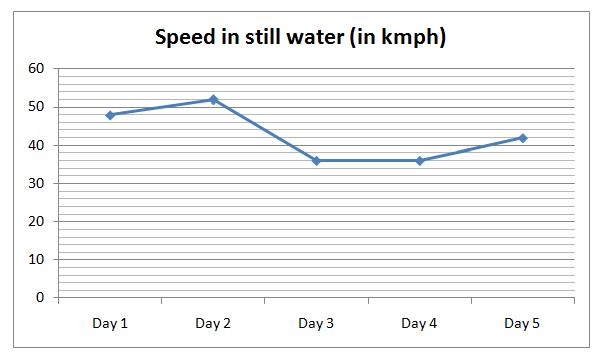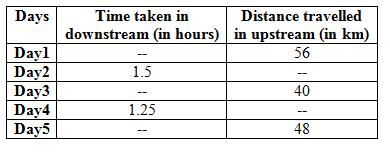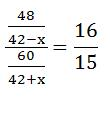# SBI Clerk Mains Quantitative Aptitude (Day-69)

## Quantitative Aptitude Materials for SBI Clerk Mains

Dear Aspirants, Our IBPS Guide team is providing new series of Quantitative Aptitude Questions for SBI Clerk Mains 2020 so the aspirants can practice it on a daily basis. These questions are framed by our skilled experts after understanding your needs thoroughly. Aspirants can practice these new series questions daily to familiarize with the exact exam pattern and make your preparation effective.

Start Quiz

Ensure Your Ability Before the ExamTake SBI Clerk 2020 Mains Free Mock Test

Data Interpretation

Direction (1-5): Study the following information carefully and answer the questions given below.

The given line graph shows the speed in still water in five days.The given table shows the time taken by downstream and the distance travelled in upstream in five different days.1) If time taken by boat on day1 in upstream is 1.4 hours which is 12% more than the time taken by the same boat on day1 in downstream, then what is the distance travelled by boat on day1 in downstream?

A) 60 km

B) 70 km

C) 84 km

D) 56 km

E) None of these

2) If the speed of stream on day3 is 12 kmph and the ratio of the time taken by the boat in upstream to downstream is 10:7, then what is the difference between the distance travelled by boat in upstream and that of in downstream on day3?

A) 12 km

B) 16 km

C) 18 km

D) 20 km

E) None of these

3) The distance travelled by the boat in downstreamon day4 and day 2 is 50 km and 84 km respectively. If the time taken in upstream on day2 and day4 is same as the time taken in downstream on same days, then find the difference between distance travelled by boat in upstream on day2 and day4?

A) 30 km

B) 28 km

C) 24 km

D) 36 km

E) None of these

4) On day5, distance travelled by boat in downstream is 25% more than the distance travelled in upstream and the ratio of time taken by boat to cover the distance in upstream to downstream is 16:15. What is the speed of stream on day5?

A) 4 kmph

B) 5 kmph

C) 6 kmph

D) 8 kmph

E) 3 kmph

Caselet

Direction (5-9): Study the following information carefully and answer the questions given below.

Arul invests Rs.9000 and the ratio of the investment of Arul to Bala is 5:3. The investment of Bala is 75% of the investment of Banu and the investment of Sharu is Rs.900 less than the investment of Esai. Divya’s investment is 70% of the investment of Esai. Total investment of all of them is Rs.45000.

5) Arul and Sharu started the business. After 5 months Hari joined with the investment of Ra.1500 more than Sharu and Arul invests Rs.1200 for his initial investment. If the total profit at the end of the business is Rs.40716, then find the profit of Hari?

A) Rs. 9744

B) Rs. 8948

C) Rs. 9064

D) Rs. 9264

E) None of these

6) Bala and Divya started the business. After 6 months Esai joined with them and Bala left the business. After 2 more months Divya left the business. If Esai invests his capital in x months and the total profit of the business is Rs.10800 and the Divya’s profit share is Rs.3150, then find the value of x?

A) 11

B) 10

C) 12

D) 8

E) 9

7) Bala, Banu and Nirmal started the business with the investment in the ratio of 3:4:6. After 7 months Nirmal left the business and Divya joined. After 2 more months Bala added Rs.1800 for his investment. At the end of year the profit of Bala and Divya is Rs.11300, then find the total profit of the business?

A) Rs.45200

B) Rs.22600

C) Rs.58600

D) Rs.29300

E) None of these

8) Bala, Esai and Banu started a business. Bala is a working partner but Esai and Banu are sleeping partner in the business. For managing the business, Bala received 10% of the total profit. If at the end of year the total profit of the business is Rs.23400, then find the difference between the share of Bala and Banu?

A) Rs.235

B) Rs.450

C) Rs.550

D) Rs.300

E) None of these

9) Sharu, Divya and Esai together started a business. After 4 months Sharu withdraw one-third of the initial investment and after another 2 months Esai withdraw one-sixth of the initial investment. If at the end of year the total profit is Rs.13900, then find the profit share of Esai?

A) Rs.4800

B) Rs.3600

C) Rs.6500

D) Rs.4200

E) 5500

Application Sums

10) Box A contains the number of apple, banana and orange in the ratio of 2:3:4 and the box B contain three fruits apple, banana and orange. If both boxes are mixed, then the ratio of the apple, banana and orange becomes 13:17:12, then find the ratio of the number of apple, banana and orange in box B?

A) 1:2:3

B) 5:7:8

C) 4:5:6

D) 6:7:8

E) Cannot be determined

Time taken in upstream = 56/(48 – x) = 1.4

(48 – x) * 1.4 = 56

x = 8

Time taken in downstream = 1.4 * 100/112 = 1.25

D/(48 + 8) = 1.25

D = 70 km

Time taken in upstream on day3 = 40/(36 – 12)  * 60 =  100 minutes

Time taken in downstream on day3 = 7/10 * 100 = 70 minutes

Distance travelled by boat on day3 in downstream = (36 + 12) * 70/60

= 56 km

Required difference = 56 – 40 = 16 km

Speed of stream on day2 = x kmph

Speed of stream on day4 = y kmph

84/(52 + x) = 1.5

x = 4 kmph

50/(36 + y) = 1.25

y = 4 kmph

Distance travelled in upstream on day2 = (52 – 4) * 1.5 = 72 km

Distance travelled in upstream on day4 = (36 – 4) * 1.25 = 40 km

Required distance = 72 – 40 = 32 km

Speed of stream = x kmph

Distance travelled by boat in downstream on day5 = 125/100 * 48 = 60 km960/(42 + x) = 720/(42 – x)

30240 + 720x = 40320 – 960x

x = 6 kmph

Direction (5-9):

Arul’s investment = Rs.9000

Bala = 3/5 * 9000 = Rs.5400

Banu = 100/75 * 5400 = Rs.7200

Esai = x

Sharu = x – 900

Divya = x * 70/100 = 7x/10

x + x – 900 + 7x/10 = 45000 – (9000 + 5400 + 7200)

2.7x = 24300

x = 9000

Esai = Rs.9000

Sharu = Rs.8100

Divya = 7/10 * 9000 = Rs.6300

The investment of Hari = 8100 + 1500 = Rs. 9600

Profit ratio of Arul, Sharu and Hari = (9000*5 + 10200*7): (8100*12): (9600*7)

= 116400: 97200: 67200

= 97: 81: 56

Profit Share of Hari = 56/234 * 40716 = Rs.9744

Profit share of Bala, Divya and Esai = 5400 * 6:6300 * 8:9000 * x

= 18:28:5x

28/(46 + 5x) = 3150/10800

28/(46 + 5x) = 7/24

96 = 46 + 5x

x = 10

Nirmal investment = 6/3 * 5400 = 10800

Profit ratio of Bala, Banu, Nirmal and Divya = (5400 * 9 + 7200 * 3):(7200 *12):(10800 * 7):(6300 * 5)

= 70200:86400:75600:31500

= 78:96:84:35

Total profit of the business = 293/113 * 11300 = Rs.29300

Profit share of Bala, Esai and Banu = 5400 * 12:9000 * 12:7200 * 12

= 3: 5: 4

For managing the business, Bala received = 23400 * (10/100) = Rs.2340

Remaining amount = 23400 – 2340 = 21060

Profit share of Bala = 2340 + (21060 * 3/12)

= Rs.7605

Profit share of Banu = 21060 * 4/12 = Rs.7020

Required difference = 7605 – 7020 = Rs.585

Profit ratio of Sharu, Divya and Esai = (8100 * 4 + 5400 * 8): 6300 * 12: (9000 *6 + 7500 * 6)

= 75600:75600:99000

= 42:42:55

Profit Share of Esai = 55/139 * 13900 = Rs.5500

Box A = 2: 3: 4

Box B = x: y: z

Mixed ratio = 13: 17: 12

We can’t find the ratio of Box B

 Check Here to View SBI Clerk Mains 2020 Quantitative Aptitude Questions Day 68 Day 67 Day 66 Click Here for SBI Clerk 2020 – Detailed Exam Notification
5 1 vote
Rating